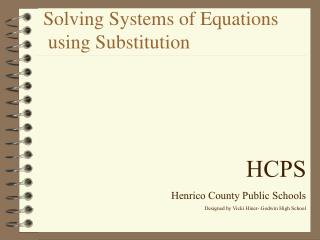DownloadDownload PresentationSolving Systems of Equations using Substitution

# Solving Systems of Equations using Substitution

Télécharger la présentation## Solving Systems of Equations using Substitution

- - - - - - - - - - - - - - - - - - - - - - - - - - - E N D - - - - - - - - - - - - - - - - - - - - - - - - - - -
##### Presentation Transcript

1. Solving Systems of Equations using Substitution HCPS Henrico County Public Schools Designed by Vicki Hiner- Godwin High School

2. Lesson Objective: • Solve systems of equations by substitution method.

3. Assignment: • pp. 450-451 #5-30 Skip 9 • Fractions on some of these, so don’t freak out.

4. Solving Systems of Equations using Substitution Steps: 1. Solve One equation for One variable( y= ; x= ; a=) 2. Substitute equation from step one into other equation (get an equation with only one variable) 3. Solve for the first variable. 4. Go back and use the found variable in step 3 to find second variable. 5. Check the solution in both equations of the system. WHAT DO WE DO???? CLICK TO SEE AN EXAMPLE

5. GIVEN EXAMPLE: y= 4x 3x+y=-21 STEP1: y=4x (Already solved for y) STEP 2: Substitute into second equation: 3x + y = -21 becomes:

6. GIVEN EXAMPLE: y= 4x 3x+y=-21 STEP1: y=4x (Already solved for y) STEP 2: Substitute into second equation: 3x + y = -21 becomes: 3x +4x =-21

7. GIVEN EXAMPLE: y= 4x 3x+y=-21 STEP 3: Solve for the variable 3x + 4x=-21 7x=-21 x=-3

8. GIVEN EXAMPLE: y= 4x 3x+y=-21 STEP 4: Solve for the other variable use x=-3 and y=4x y=4x and x = -3 therefore: y=4(-3) or y = -12 Solution to the system is (-3,-12)

9. GIVEN EXAMPLE: y= 4x 3x+y=-21 Check solution ( -3,-12) y=4x -12=4(-3) -12=-12 3x+y=-21 3(-3)+(-12)=-21 -9+(-12)=-21 -21=-21

10. Solving Systems of Equations using Substitution Review Steps --Questions? Steps: 1. Solve One equation for One variable( y= ; x= ; a=) 2. Substitute equation from step one into other equation (get an equation with only one variable) 3. Solve for the first variable. 4. Go back and use the found variable in step 3 to find second variable. 5. Check the solution in both equations of the system.

11. GIVEN EXAMPLE: x + y=10 5x - y=2 STEP1: Solve for y x + y = 10 y = -x +10 STEP 2: Substitute into second equation: 5x - y = 2 becomes:

12. GIVEN EXAMPLE: x + y=10 5x - y=2 STEP1: Solve for Y x + y = 10 y = -x +10 STEP 2: Substitute into second equation: 5x - y = 2 becomes: 5x - (-x+10) = 2

13. GIVEN EXAMPLE: x + y=10 5x - y=2 STEP 3: Solve for the variable 5x-(-x+10)=2 5x+x-10=2 6x-10=2 6x=12 x=2

14. GIVEN EXAMPLE: x + y=10 5x - y=2 STEP 4: Solve for the other variable use x=2 and x+y=10 x=2 and x+y = 10 therefore: 2+y=10 and y = 8 Solution to the system is (2,8)

15. GIVEN EXAMPLE: x + y=10 5x - y=2 Check solution (2,8) 5x-y=2 5(2)-(8)=2 10-8=2 2=2 x + y=10 2+8=10 10=10